This example shows calculation of the radiation efficiency of the antenna and antenna arrays in Antenna Toolbox. The radiation efficiency of an antenna is defined as the ratio of the outward radiated power by the antenna to the input power fed to the excitation port of the antenna. The power loss due to the port impedance mismatch is not considered here.

The input power fed to the antenna can be written as

${P}_{in}=\frac{1}{2}{V}_{in}{I}_{in}^{*}.$ (1)

Here, the input voltage and input current are represented by ${V}_{in}$ and ${I}_{in}$, respectively. The complex conjugation of the input current is ${I}_{in}^{*}$. The power ${P}_{rad}$ radiated by the antenna can be found by integrating the radiation intensity ($U\left(\theta ,\varphi \right)$) over the infinite radiation sphere (${S}_{\infty }$) as

${P}_{rad}={\int }_{{S}_{\infty }}U\left(\theta ,\varphi \right)sin\theta d\theta d\varphi .$ (2)

The azimuth and elevation angles are denoted by $\varphi$ and $\theta$, respectively. The radiation efficiency (${\eta }_{r}\right)$ is defined as

${\eta }_{r}=\frac{{P}_{rad}}{{P}_{in}}.$ (3)

The difference between the input power and the radiated power is due to the conduction loss in the metal-only antennas and due to both the conduction loss and dielectric loss in the metal-dielectric antennas. The radiation efficiency is also alternately defined as the gain and directivity of the antenna. In other words,

$G\left(\theta ,\varphi \right)={\eta }_{r}D\left(\theta ,\varphi \right).$ (4)

For ideal lossless antenna, the radiation efficiency (${\eta }_{r}$) is 1.

### Metal only Antenna

This example considers a yagi-uda antenna with the same dimensions as given in .

### Create Geometry

Create the geometry of the yagi-uda antenna with two director elements having the length values of 131.9 mm and 126.5 mm, respectively. The spacing dimensions for the directors are 65.95 mm and 80.34 mm. The length of the Exciter is 139.1 mm. The length and spacing values of the reflector are 141.5 mm and 88.13 mm, respectively. In , all the elements were thin wires with the radius of 0.6745 mm. However, equivalent strip modelling is followed using `cylinder2strip` function.

```d=design(dipole,1e9); radius=6.7450e-04; %Radius of thin wires d.Width=cylinder2strip(radius); %Converting into equivalent stripwidth d.Length=139.1e-03; d.TiltAxis=[0 1 0]; d.Tilt=-90; ant=design(yagiUda,1e9); ant.Exciter=d; ant.NumDirectors=2; ant.DirectorLength=[131.9e-03;126.5e-03]; ant.DirectorSpacing=[65.95e-03;80.34e-03]; ant.ReflectorLength=141.5e-03; ant.ReflectorSpacing=88.13e-03;```

### Visualize Antenna

Visualize of the default perfect electrically conducting (PEC) antenna whose default conductivity and thickness values are infinite and zero, respectively.

```figure; show(ant)```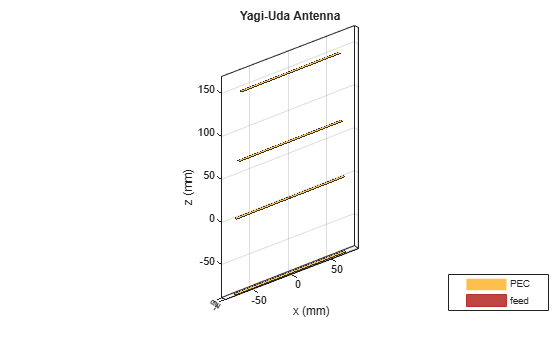The show function provides the name of the conductor and the feed location using different colors in the above figure.

### Visualize Radiation Efficiency of PEC Antenna

Plots the radiation efficiency of the PEC yagi-uda antenna using `efficiency` function within the frequency range of 0.5 GHz to 1.5 GHz with 31 sampling points. As the PEC antenna has no loss, it shows the radiation efficiency as 1 over the frequency range here.

```f=linspace(0.5e9, 1.5e9, 31); efficiency(ant,f)```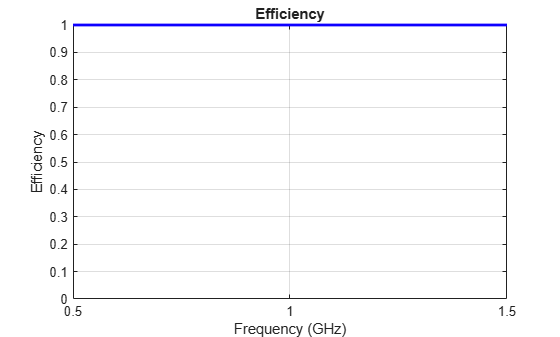### Visualize Directivity of PEC antenna

It provides the directivity of the PEC yagi-uda antenna at a frequency of 1 GHz. Due to the absence of loss, the directivity and gain will be same in the PEC antenna.

```figure; pattern(ant, 1e9)```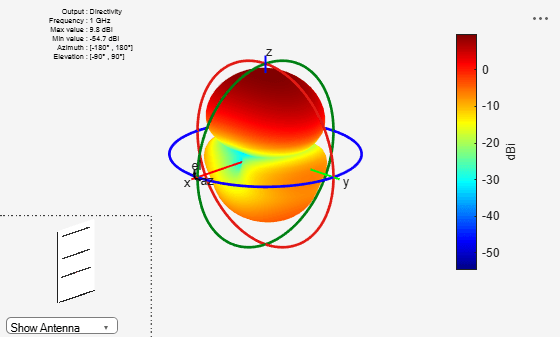### Use Copper Metal for Antenna Design

Set the conductor as copper from the metal catalog of the Antenna Toolbox. Modify the conductivity of the finite metal yagi-uda antenna using properties of metal object.

### Use Copper Metal for Antenna Design

```ant.Exciter.Conductor=metal('Copper');%chosing the conductor from the metal catalog ant.Exciter.Conductor.Conductivity=1e5;%same value as of the reference paper```

### Specify Metal Properties of Exciter

```ant.Exciter.Conductor=metal('Copper');%chosing the conductor from the metal catalog ant.Exciter.Conductor.Conductivity=1e5;%same value as of the reference paper```

### Specify Metal Properties of Conductor

```ant.Conductor=metal('Copper');%chosing the conductor from the metal catalog ant.Conductor.Conductivity=1e5;%same value as of the reference paper```

It manually modifies the conductivity and thickness of the finite metal yagi-uda antenna.

```ant.Exciter.Conductor.Thickness=700*1e-6; ant.Conductor.Thickness=700*1e-6;```

### Visualize Finite Metallic Antenna

Visualize the metallic yagi-uda antenna using show function.

```figure; show(ant)```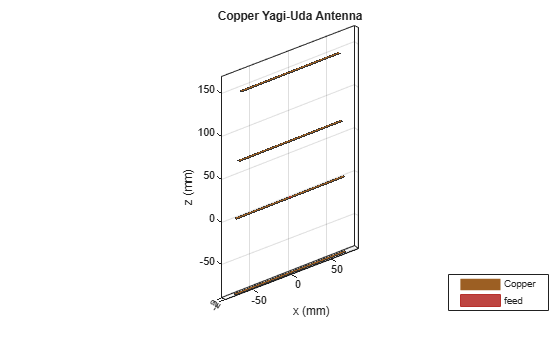### Plot Radiation Efficiency of Finite Metallic Antenna

Plots the radiation efficiency visualization of the metallic yagi-uda antenna at the frequency range of 0.5 GHz to 1.5 GHz.

```f=linspace(0.5e9, 1.5e9, 31); figure; efficiency(ant,f)```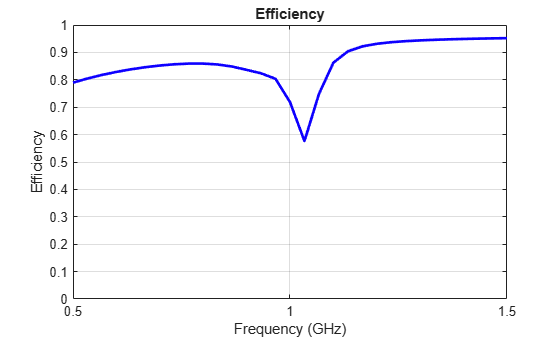### Plot Gain Pattern of Finite Metallic Antenna

Plots the gain pattern of the metallic yagi-uda antenna at a frequency of 1 GHz.

```figure pattern(ant,1e9)```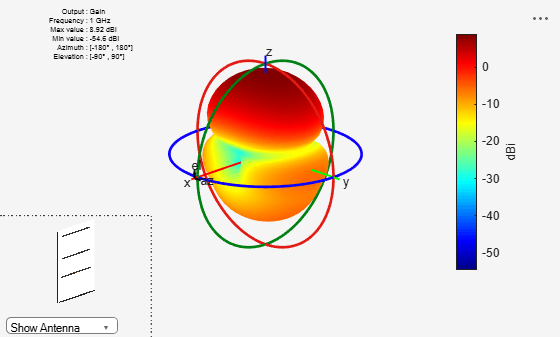It can be noted from the above comparisons that the gain of the antenna is reduced by 0.86 dB due to finite conduction loss. The efficiency value is closely matching with the analytical results of .

### Metal-dielectric Antenna

This example considers a microstrip-patch antenna from . In , the numerical analysis was done using the Finite-difference time-domain (FDTD) technique. However, the antenna is analyzed here using the method of moment (MoM) based solver of the Antenna Toolbox.

### Create Geometry

Creates the geometry of the microstrip-patch antenna with PEC conductor and lossy substrate of 1.57 mm thickness.

```f=1.59e9; %solution frequency lambda=3e8/f; d = dielectric('FR4'); %Selecting the substrate from the dielectric catalog d.EpsilonR=4.36; d.LossTangent=2/100;%Indicates the lossy substrate ant = patchMicrostrip('Substrate',d); ant.Height=1.57e-3; ant.Substrate.Thickness=1.57e-3; ant.Length=45e-3; ant.Width=45e-3; ant.GroundPlaneLength=20e-2; ant.GroundPlaneWidth=13.5e-2; ant.FeedOffset= [20e-3 0]; ant.FeedWidth=lambda/200;```

### Manual Meshing of Microstrip Patch Antenna

Mesh the antenna manually by using the maximum edgelength of the RWG basis functions as lambda/20 where the free-space wavelength at the solution frequency of 1.5 GHz is lambda.

```figure; mesh(ant,'MaxEdgeLength',lambda/20)```### Visualize Microstrip Patch Antenna

Visualize the schematic show of the antenna with PEC as metal and lossy substrate.

```figure; show(ant)```### Calculate Radiation Efficiency of Microstrip Antenna with PEC Metal and Lossy Substrate

Calculates the efficiency in absolute and logarithmic values of the radiation efficiency of the antenna with PEC as metal and lossy substrate. As the metal is PEC, only loss is due to the lossy substrate.

`E1=efficiency(ant,f)`
```E1 = 0.3108 ```
`E1_log=10*log10(E1)`
```E1_log = -5.0757 ```

### Plot Directivity of Microstrip Antenna with PEC Metal and Lossy Substrate

Plots the directivity of the microstrip antenna with PEC metal and lossy substrate using pattern function. The directivity does not depend on the conduction and dielectric losses.

```figure pattern(ant,f,'Type', 'Directivity')```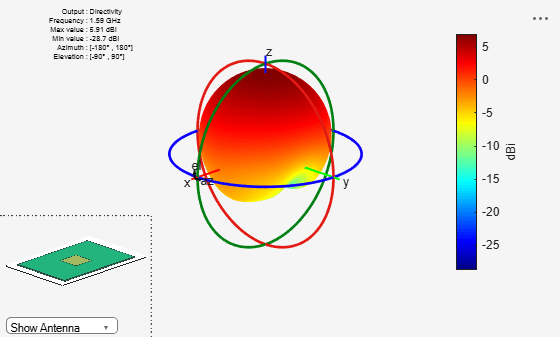### Plot Gain of Microstrip Antenna with PEC Metal and Lossy Substrate

Plots the gain of the microstrip antenna with PEC metal and lossy substrate. Here, the gain value is less than the directivity value due to the dielectric loss. The difference of the gain and directivity values matches closely with the log value of the radiation efficiency, i.e., $E{1}_{log}$.

```figure; pattern(ant,f,'Type', 'Gain')```### Impedance of Microstrip Antenna with PEC Metal and Lossy Substrate

Plots the impedance variation of the microstrip antenna with PEC metal and lossy substrate in the frequency range of 1 GHz to 4 GHz.

```f1=linspace(1e9,4e9,31); figure impedance(ant,f1)```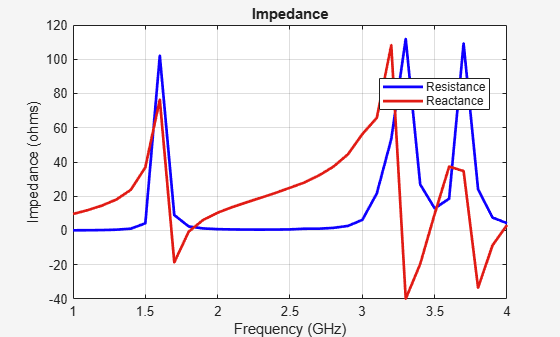### Return Loss of Microstrip Antenna with PEC Metal and Lossy Substrate

Plots the return loss variation of the microstrip antenna with PEC metal and lossy substrate.

```figure; s1=sparameters(ant,f1,50); rfplot(s1);```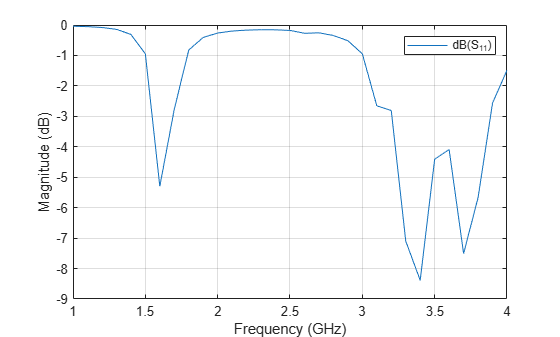### Change Conductor Properties of Microstrip Antenna

Set conductor of antenna as lossy metal. Use metal object to change conductor to copper metal.

`ant.Conductor=metal('Copper');`

### Visualize Microstrip Antenna with Lossy Metal and Lossy Substrate

Visualize of the microstrip antenna with Copper metal and FR4 substrate.

```figure; show(ant)```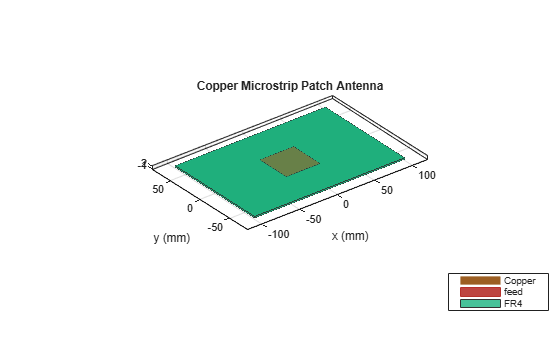### Calculate Radiation Efficiency of Microstrip Antenna with Lossy Metal and Lossy Substrate

Calculates the radiation efficiency in absolute and logarithmic values of the microstrip antenna with Copper as metal and lossy FR4 as substrate. Due to the

conduction loss in addition to the dielectric loss, the radiation efficiency is reduced.

`E2=efficiency(ant,f)`
```E2 = 0.2255 ```
`E2_log=10*log10(E2)`
```E2_log = -6.4692 ```

### Plot Gain of Microstrip Antenna with Lossy Metal and Lossy Substrate

Plot the gain of the microstrip antenna with Copper metal and FR4 substrate.

```figure; pattern(ant,f,'Type', 'Gain')```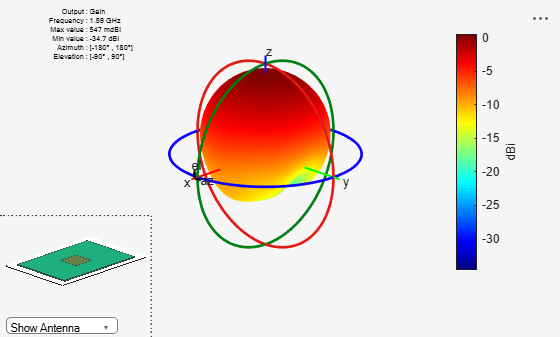Here, the gain value is less than the directivity value due to both the conduction and dielectric losses. The difference of the gain and directivity matches closely with the log value of the radiation efficiency, i.e., $E{2}_{log}$.

### Conclusion

Thus, the radiation efficiencies computed using the Antenna Toolbox for both the metallic and metal-dielectric antennas are found to match closely as reported in the references which used different analytic  or numerical techniques .

### References

 Shahpari, Morteza, and David V. Thiel. "Fundamental limitations for antenna radiation efficiency," IEEE Transactions on Antennas and Propagation, Vol. 66, No. 8, 2018.

 Ph. Leveque, A. Reineix and B. Jecko, “Modelling of Dielectric Losses in Microstrip Patch Antennas: Application of FDTD Method”, Electronics Letters, Vol. 28, No. 6, March 1992.